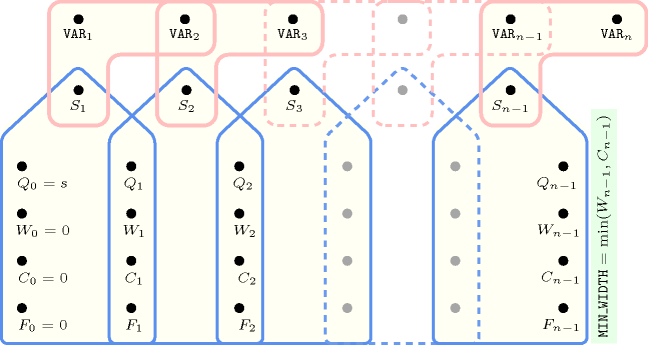## 5.260. min_width_peak

Origin
Constraint

$\mathrm{𝚖𝚒𝚗}_\mathrm{𝚠𝚒𝚍𝚝𝚑}_\mathrm{𝚙𝚎𝚊𝚔}\left(\mathrm{𝙼𝙸𝙽}_\mathrm{𝚆𝙸𝙳𝚃𝙷},\mathrm{𝚅𝙰𝚁𝙸𝙰𝙱𝙻𝙴𝚂}\right)$

Synonym

$\mathrm{𝚖𝚒𝚗}_\mathrm{𝚋𝚊𝚜𝚎}_\mathrm{𝚙𝚎𝚊𝚔}$.

Arguments
 $\mathrm{𝙼𝙸𝙽}_\mathrm{𝚆𝙸𝙳𝚃𝙷}$ $\mathrm{𝚍𝚟𝚊𝚛}$ $\mathrm{𝚅𝙰𝚁𝙸𝙰𝙱𝙻𝙴𝚂}$ $\mathrm{𝚌𝚘𝚕𝚕𝚎𝚌𝚝𝚒𝚘𝚗}\left(\mathrm{𝚟𝚊𝚛}-\mathrm{𝚍𝚟𝚊𝚛}\right)$
Restrictions
 $\mathrm{𝙼𝙸𝙽}_\mathrm{𝚆𝙸𝙳𝚃𝙷}\ge 0$ $\mathrm{𝙼𝙸𝙽}_\mathrm{𝚆𝙸𝙳𝚃𝙷}\le |\mathrm{𝚅𝙰𝚁𝙸𝙰𝙱𝙻𝙴𝚂}|-2$ $\mathrm{𝚛𝚎𝚚𝚞𝚒𝚛𝚎𝚍}$$\left(\mathrm{𝚅𝙰𝚁𝙸𝙰𝙱𝙻𝙴𝚂},\mathrm{𝚟𝚊𝚛}\right)$
Purpose

Given a sequence $\mathrm{𝚅𝙰𝚁𝙸𝙰𝙱𝙻𝙴𝚂}$ constraint $\mathrm{𝙼𝙸𝙽}_\mathrm{𝚆𝙸𝙳𝚃𝙷}$ to be fixed to the width of the smallest peak, or to 0 if no peak exists.

Example
 $\left(5,〈4,4,2,2,3,5,5,6,3,1,1,2,2,2,2,2,2,1〉\right)$ $\left(5,〈4,6,7,9,8,5,4〉\right)$ $\left(0,〈4,4,2,0,0,4,5〉\right)$

The first $\mathrm{𝚖𝚒𝚗}_\mathrm{𝚠𝚒𝚍𝚝𝚑}_\mathrm{𝚙𝚎𝚊𝚔}$ constraint holds since the sequence $442235563112222221$ contains two peaks of respective width 5 and 6 (see Figure 5.260.1) and since its argument $\mathrm{𝙼𝙸𝙽}_\mathrm{𝚆𝙸𝙳𝚃𝙷}$ is fixed to the smallest value 5.

##### Figure 5.260.1. Illustration of the first example of the Example slot: a sequence of eighteen variables ${V}_{1}$, ${V}_{2}$, ${V}_{3}$, ${V}_{4}$, ${V}_{5}$, ${V}_{6}$, ${V}_{7}$, ${V}_{8}$, ${V}_{9}$, ${V}_{10}$, ${V}_{11}$, ${V}_{12}$, ${V}_{13}$, ${V}_{14}$, ${V}_{15}$, ${V}_{16}$, ${V}_{17}$, ${V}_{18}$ respectively fixed to values 4, 4, 2, 2, 3, 5, 5, 6, 3, 1, 1, 2, 2, 2, 2, 2, 2, 1 and its two peaks of width 5 and 6.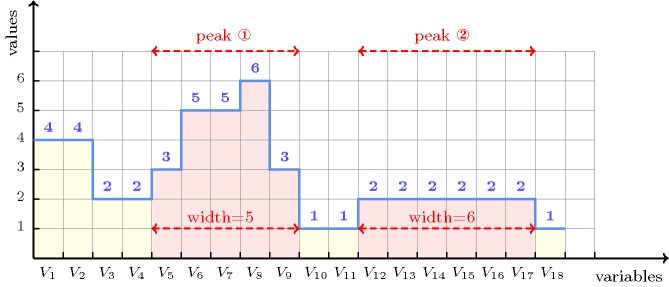Typical
 $\mathrm{𝙼𝙸𝙽}_\mathrm{𝚆𝙸𝙳𝚃𝙷}>1$ $|\mathrm{𝚅𝙰𝚁𝙸𝙰𝙱𝙻𝙴𝚂}|>2$
Symmetries
• Items of $\mathrm{𝚅𝙰𝚁𝙸𝙰𝙱𝙻𝙴𝚂}$ can be reversed.

• One and the same constant can be added to the $\mathrm{𝚟𝚊𝚛}$ attribute of all items of $\mathrm{𝚅𝙰𝚁𝙸𝙰𝙱𝙻𝙴𝚂}$.

Arg. properties

Functional dependency: $\mathrm{𝙼𝙸𝙽}_\mathrm{𝚆𝙸𝙳𝚃𝙷}$ determined by $\mathrm{𝚅𝙰𝚁𝙸𝙰𝙱𝙻𝙴𝚂}$.

Counting
 Length ($n$) 2 3 4 5 6 7 8 Solutions 9 64 625 7776 117649 2097152 43046721

Number of solutions for $\mathrm{𝚖𝚒𝚗}_\mathrm{𝚠𝚒𝚍𝚝𝚑}_\mathrm{𝚙𝚎𝚊𝚔}$: domains $0..n$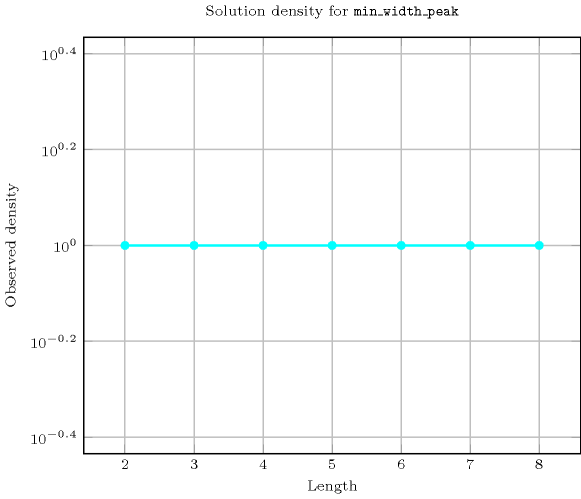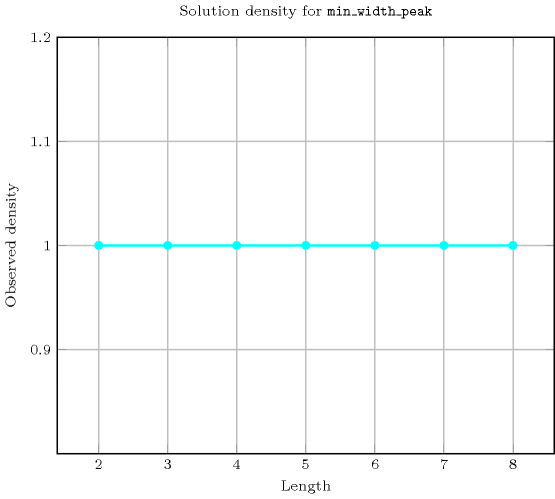Length ($n$)2345678
Total9646257776117649209715243046721
 Parameter value

095029517921108869498439791
1-14230320556637117439826327058
2--1002100284204249289363060
3---679170242687223413256
4----44801304522345982
5-----29154968946
6------188628

Solution count for $\mathrm{𝚖𝚒𝚗}_\mathrm{𝚠𝚒𝚍𝚝𝚑}_\mathrm{𝚙𝚎𝚊𝚔}$: domains $0..n$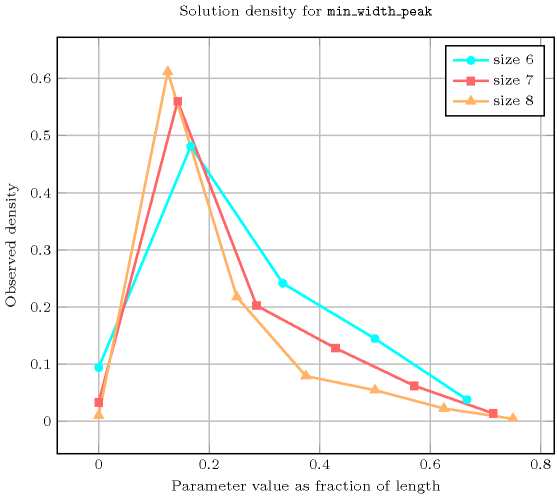Keywords
Automaton

Figure 5.260.2 depicts the automaton associated with the $\mathrm{𝚖𝚒𝚗}_\mathrm{𝚠𝚒𝚍𝚝𝚑}_\mathrm{𝚙𝚎𝚊𝚔}$ constraint. To each pair of consecutive variables $\left({\mathrm{𝚅𝙰𝚁}}_{i},{\mathrm{𝚅𝙰𝚁}}_{i+1}\right)$ of the collection $\mathrm{𝚅𝙰𝚁𝙸𝙰𝙱𝙻𝙴𝚂}$ corresponds a signature variable ${S}_{i}$. The following signature constraint links ${\mathrm{𝚅𝙰𝚁}}_{i}$, ${\mathrm{𝚅𝙰𝚁}}_{i+1}$ and ${S}_{i}$: $\left({\mathrm{𝚅𝙰𝚁}}_{i}<{\mathrm{𝚅𝙰𝚁}}_{i+1}⇔{S}_{i}=0\right)\wedge \left({\mathrm{𝚅𝙰𝚁}}_{i}={\mathrm{𝚅𝙰𝚁}}_{i+1}⇔{S}_{i}=1\right)\wedge \left({\mathrm{𝚅𝙰𝚁}}_{i}>{\mathrm{𝚅𝙰𝚁}}_{i+1}⇔{S}_{i}=2\right)$.

##### Figure 5.260.2. Automaton of the $\mathrm{𝚖𝚒𝚗}_\mathrm{𝚠𝚒𝚍𝚝𝚑}_\mathrm{𝚙𝚎𝚊𝚔}$ constraint: the start of the first potential peak is discovered while triggering the transition from $s$ to $j$, the top of a peak is discovered while triggering the transition from $j$ to $k$, the end of a peak and the start of the next potential peak are discovered while triggering the transition from $k$ to $j$; the counters $W$, $C$ and $F$ respectively stand for min_width, current and first.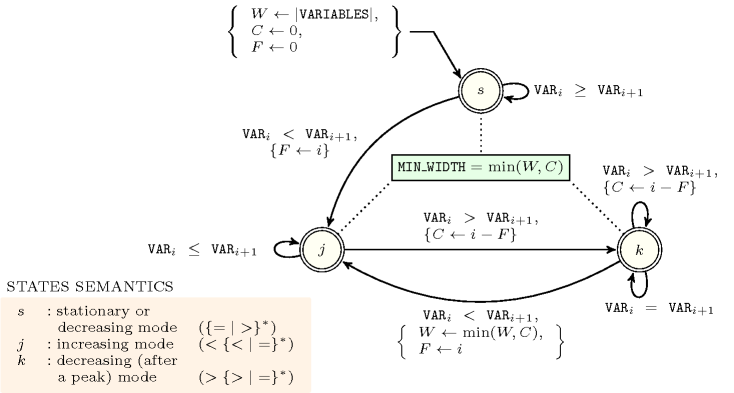##### Figure 5.260.3. Glue matrix associated with the automaton of the $\mathrm{𝚖𝚒𝚗}_\mathrm{𝚠𝚒𝚍𝚝𝚑}_\mathrm{𝚙𝚎𝚊𝚔}$ constraint, where $n$ stands for $|\mathrm{𝚅𝙰𝚁𝙸𝙰𝙱𝙻𝙴𝚂}|$
 $s$ $\left({\left\{>|=\right\}}^{*}\right)$ $j$ $\left(<{\left\{<|=\right\}}^{*}\right)$ $k$ $\left(>{\left\{>|=\right\}}^{*}\right)$ $s$ $\left({\left\{>|=\right\}}^{*}\right)$ 0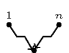$\stackrel{←}{\mathrm{𝙼𝙸𝙽}_\mathrm{𝚆𝙸𝙳𝚃𝙷}}$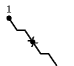$\stackrel{←}{\mathrm{𝙼𝙸𝙽}_\mathrm{𝚆𝙸𝙳𝚃𝙷}}$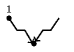$j$ $\left(<{\left\{<|=\right\}}^{*}\right)$ $\stackrel{\to }{\mathrm{𝙼𝙸𝙽}_\mathrm{𝚆𝙸𝙳𝚃𝙷}}$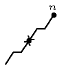$min\left(\begin{array}{c}\stackrel{\to }{W},\\ n-\stackrel{\to }{F}-\stackrel{←}{F},\\ \stackrel{←}{W}\end{array}\right)$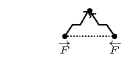$min\left(\begin{array}{c}\stackrel{\to }{\mathrm{𝙼𝙸𝙽}_\mathrm{𝚆𝙸𝙳𝚃𝙷}},\\ n-\stackrel{\to }{F}-\stackrel{←}{F},\\ \stackrel{←}{\mathrm{𝙼𝙸𝙽}_\mathrm{𝚆𝙸𝙳𝚃𝙷}}\end{array}\right)$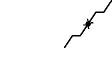$k$ $\left(>{\left\{>|=\right\}}^{*}\right)$ $\stackrel{\to }{\mathrm{𝙼𝙸𝙽}_\mathrm{𝚆𝙸𝙳𝚃𝙷}}$$min\left(\begin{array}{c}\stackrel{\to }{\mathrm{𝙼𝙸𝙽}_\mathrm{𝚆𝙸𝙳𝚃𝙷}},\\ n-\stackrel{\to }{F}-\stackrel{←}{F},\\ \stackrel{←}{\mathrm{𝙼𝙸𝙽}_\mathrm{𝚆𝙸𝙳𝚃𝙷}}\end{array}\right)$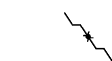$min\left(\begin{array}{c}\stackrel{\to }{\mathrm{𝙼𝙸𝙽}_\mathrm{𝚆𝙸𝙳𝚃𝙷}},\\ \stackrel{←}{\mathrm{𝙼𝙸𝙽}_\mathrm{𝚆𝙸𝙳𝚃𝙷}}\end{array}\right)$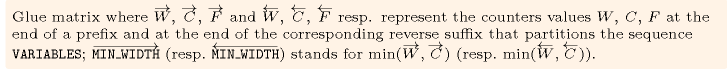##### Figure 5.260.4. Illustrating the use of the state pair $\left(j,j\right)$ of the glue matrix for linking $\mathrm{𝙼𝙸𝙽}_\mathrm{𝚆𝙸𝙳𝚃𝙷}$ with the counters variables obtained after reading the prefix $4,6,7,9$ and corresponding suffix $9,8,5,4$ of the sequence $4,6,7,9,8,5,4$; note that the suffix $9,8,5,4$ (in pink) is proceed in reverse order; the left (resp. right) table shows the initialisation (for $i=0$) and the evolution (for $i>0$) of the state of the automaton and its counters $W$, $C$ and $F$ upon reading the prefix $4,6,7,9$ (resp. the reverse suffix $4,5,8,9$).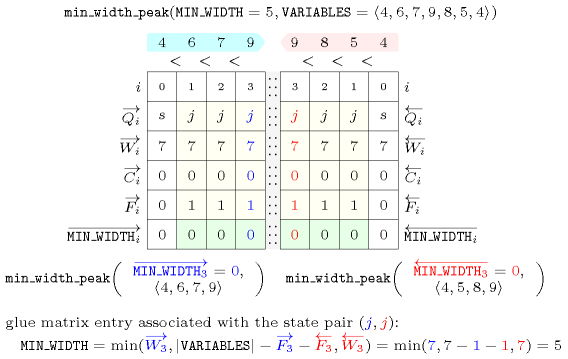##### Figure 5.260.5. Hypergraph of the reformulation corresponding to the automaton of the $\mathrm{𝚖𝚒𝚗}_\mathrm{𝚠𝚒𝚍𝚝𝚑}_\mathrm{𝚙𝚎𝚊𝚔}$ constraint